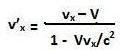## Saturday, November 21, 2009

### Irodov Problem 1.359

a) The position of the particles as seen in the laboratory frame of reference are given by x1 = v1t and x2 = -v2t. The relative velocity is thus simple the time derivative of x1-x2 given by v1+v2.

b) For this part of the problem we could use the result obtained from Problem 1.358 namely,To obtain the velocity of particle 2 as seen from particle 1 we have to substitute V=v1 and vx = -v2 in the above equation. Hence, we obtain,